Posted on

## Homework Help Complex Problems – Moving Deeper into Concepts

As you move deeper into each textbook (Algebra, Geometry, Algebra 2 w/Trig, Calculus, etc), the problems will contain several concepts you’ve learned earlier – all in one problem.  This complexity can make it difficult to find the “how-to” or examples in the book to help us understand how to work it.

Recently, we received this question from our student support page: “There doesn’t seem to be any examples or teaching that I can find that helps me solve this problem.”

This is a common question – not this particular problem, but in general, as the problems develop into including several simple concepts stacked into compound calculations. However, the explanations are there, they just may be back a few, or several, chapters.

For example, Jacobs Algebra Chapter 12 Summary and Review Problem 14h.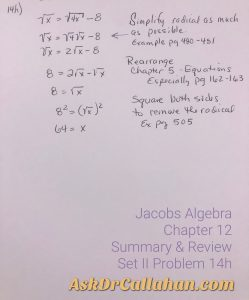Concepts include but are not limited to:

• Chapter 12: Square Roots. Simplify radical as much as possible. Example of this step page 480-481
• Chapter 5: Equations in One Variable. Specifically for this review problem, Equivalent Equations (Lesson 3) page 162-163.
• Chapter 12: Square Roots: Radical Equations. Page 505 has examples of squaring both sides to eliminate the radical.

Math builds on itself. As you learn more and more concepts, the problems reach back and build on concepts and analyzing skills learned earlier in the book or even in an earlier course. These complex problems can be hard to find “how-to” when we just can’t see it! The solutions manual is a good resource to help with steps, but sometimes even with those steps, we need to see where it was explained or taught.

We are here to help! Send us your homework questions and let us help. Go to our support page for help. Be sure to include the 5 points below. These points make sure you’ve told us what we need to know to help you.

## Be sure to tell us:

1. the course,
2. the chapter,
3. the lesson,
4. the problem,
5. YOUR issue as best as you can explain it.

We love to help.

Posted on

## Jacobs Geometry Chapter 15 Lesson 1 Problem 57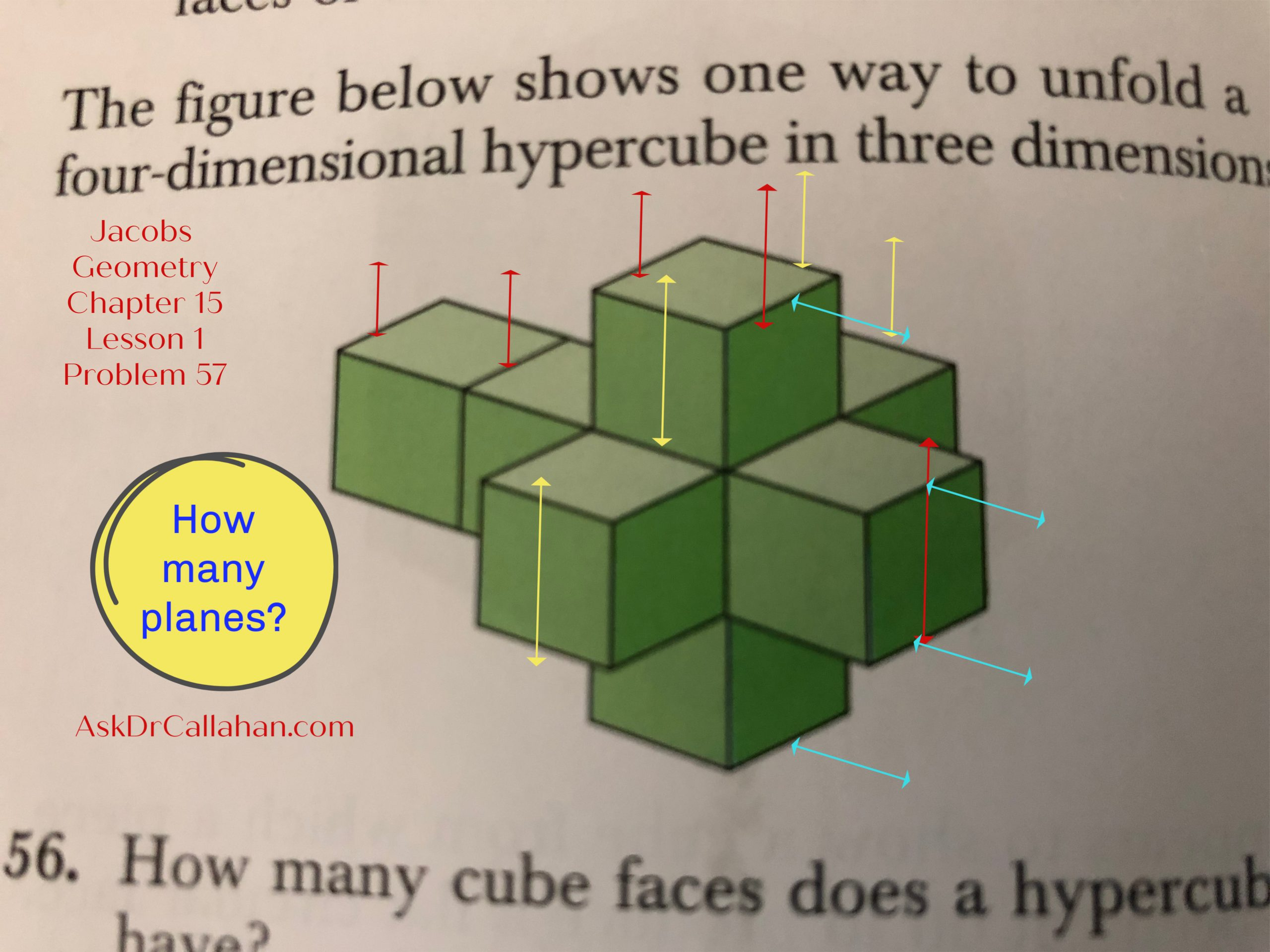In Jacobs Geometry Chapter 15 Lesson 1 problem 57, we are asked,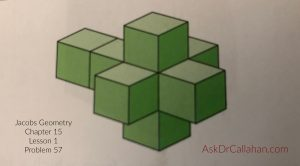“In how many planes do the square faces of the cube faces of the unfolded hypercube lie?”

The Solutions Manual says 13 planes. Let’s see how they got that number.

Imagine the red, yellow, and blue lines drawn on the hypercube as planes.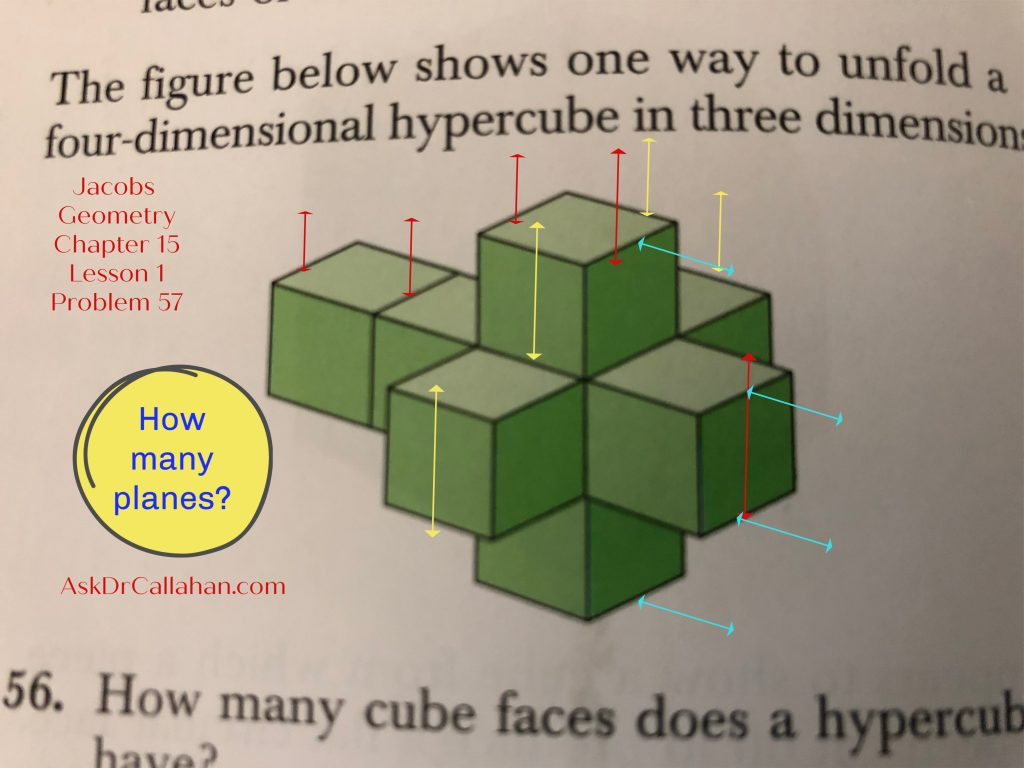Counting vertical planes from right to left: marked in red we have 5.

Counting vertical planes from front to back: marked in yellow we have 4.

Counting horizontal planes from bottom to top: marked in blue we have 4.

5 + 4 + 4 = 13 planes.

Need help with your homework problem? AskDrCallahan video instruction students can submit questions to support@askdrcallahan.com or through our website form on the student help page. Please always include the course, chapter, lesson, and problem number. Any description of your struggle is also very helpful so we get directly to your issue.  We love to help.

Posted on

## Geometry: Note on Missing Chapters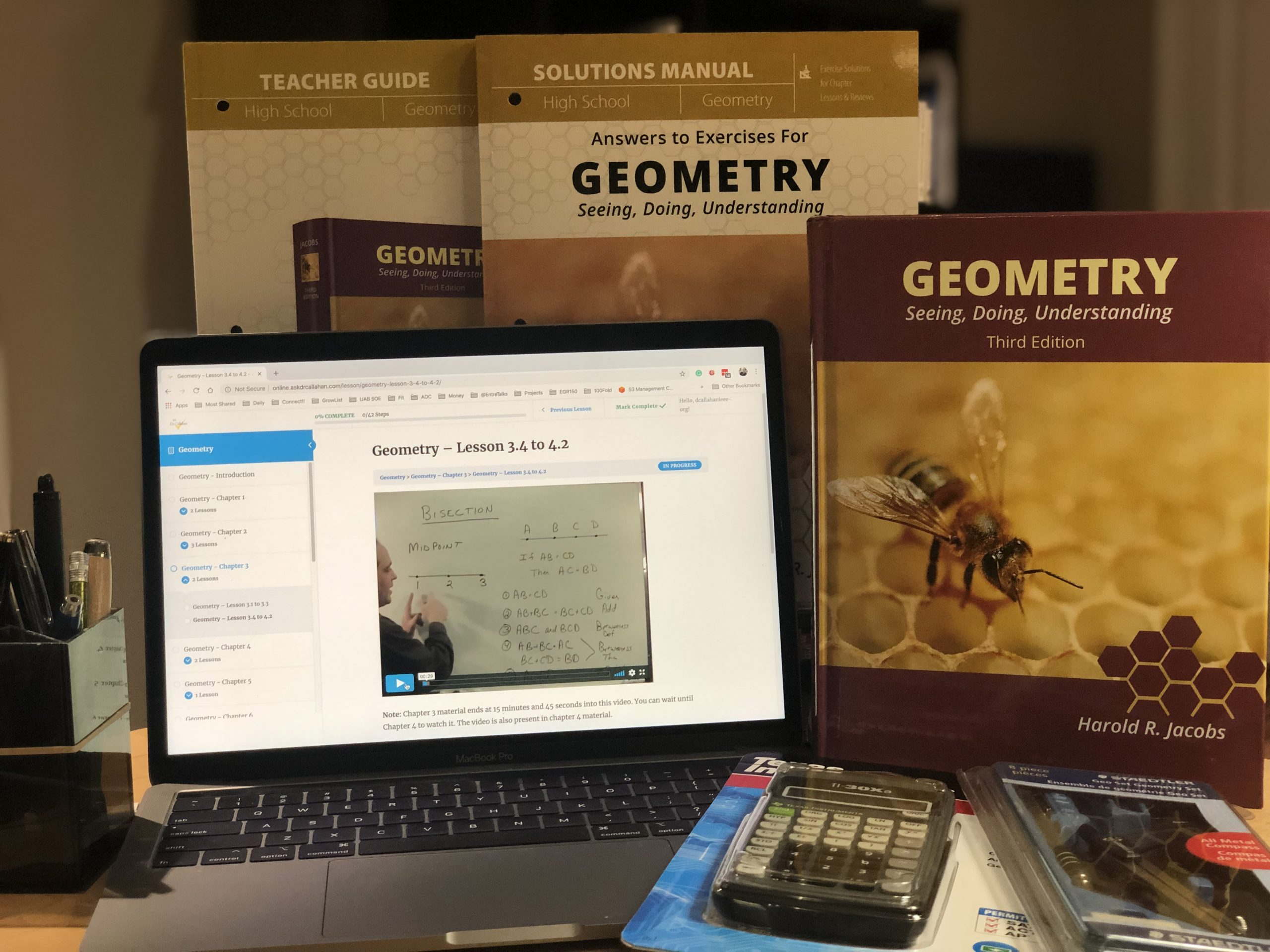## Geometry Course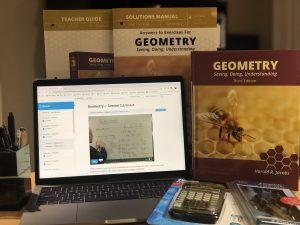Chapters 13 and 16 were originally omitted from our Geometry course for time constraints. The concepts in those chapters are advanced, though not difficult. Students will not likely see them on the ACT/SAT or even in college, except for specialized fields. When needed in a college track, they will be covered anew. They are often skipped at the high school level.

## Geometry Chapter 13 and Chapter 16However, we now recommend Chapter 13 be covered. Chapter 14 briefly refers to concepts introduced there and some students need the information. Others will be okay just referring back. Most of our students still skip chapter 13. We still consider Chapter 16 optional for everyone.
We have lectures for both chapters now on our website for FREE.
Remember that our video instruction, online or DVD, can be used with any printing of the 3rd Edition of Jacobs Geometry.
Posted on

## Algebra Chapter 16 Lesson 2 Set 1 Problem #3

This is an error in the book by Master Books (ISBN: 978-0890519851) – the book with the blue cover showing the world.

The problem is discussing hens and rabbits with a total of 30 heads and 86 feet.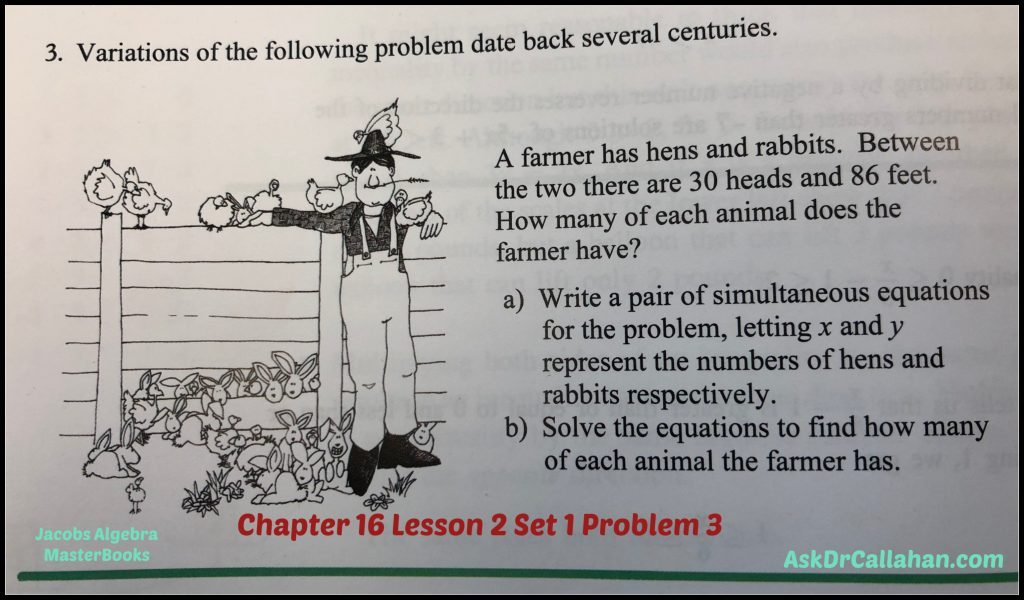ERROR: The solution manual is referring to the wrong problem which is related to money.

Here is how to solve. Simultaneous equations are covered in Chapter 7. So if you forget how to solve these, refer back to chapter 7.

heads = # hens + # rabbits

feet = 2 x # hens + 4x number of rabbits

So let h be the number of hens and r be the number of rabbits.

We can re-write the above as

heads = 30 = h + r

feet = 86 = 2h + 4r

30=h+r

So r = 30-h

Substitute this equation into the second equation to get

86 = 2h + 4r

86 = 2h + 4(30-h) = 2h + 120 – 4h = -2h +120

Rearrange to get

2h = 120-86 = 34

h = 17 (number of hens)

Now we can find the rabbits are

R = 30-h = 30-17 = 13

Checking our work

13 + 17 = 30 (total number of heads)

2 x 17 + 4 x 13 = 86 which are the number of feet.

Correct!

Posted on

## Algebra Ch13 Test A Problem #13

Question from Grace:

We are using this test A as a practice test. We can’t figure out how to solve this.

Grace,

To solve this one, you take the square root of each side

+/- SQRT ( 16x^2 + 8x +1 ) = +/- SQRT (9)

The left side needs a little work to do this. Best to see if we can factor
16x^2 + 8x +1

As a hint, you know your factors would need to be the same since you are taking a square root.

So start with the first term 16x^2 – square root of it is 4x. So try that

(4x + ___) (4x + ___)

Then the last term is 1. So the obvious thing that can be multiplied by itself to get 1 is 1. So try

(4x + 1) (4x + 1)

Now when I multiply is out I get

16x^2 + 4x + 4x + 1 = 16x^2 + 8x +1

So that works!!

Now back to the problem

+/- SQRT ( 16x^2 + 8x +1 ) = +/- SQRT (9)

+/- SQRT ((4x + 1) (4x + 1)) = +/- SQRT (9)

Using the + we have
(4x + 1) = 3
And 4x = 2 so x = 1/2 is one answer

Using the – we have
(4x + 1) = -3
4x+1 = -3
4x = -4
x = -1

Posted on

## Algebra Ch10.2 #3Error in Solutions Manual

The Solutions Manual has the answer from the older version of the book (which used a flag of 104 x 235. Flags have grown since then. 😉)

Just FYI – the older flag was flown in Detroit on the side of the J.L. Hudson Store – which no longer exists.
See it here: Worlds Largest Flag

Answer to a) Perimeter, 1520 feet; Area, 128,775 square feet
Answer to b) Perimeter, 1524 feet; Area, 129,536 square feet
Answer to c) Perimeter, (1520 + 4x) feet; Area, (128,775 + 760x + x^2)

Posted on

## Geometry Ch11 test #7b

Question from Anya
Course: Geometry
Chapter/Lesson/Problem11 test7b
Issue: I’m supposed to find Angle APM. To find the angle, I used tan APM=20/70, which gives 15.9 degrees. The solutions manual used sin APM=20/70, which gives 16.6 degrees. Sine is opposite/hypotenuse. However, it seems to me that the given measurements of the problem are opposite side (20) to the adjacent side (70). Which is correct? Thank you!
Yes, you are correct. That should be tangent as you stated.
tan(APM) = 20/70
Posted on

## Algebra Ch5.6 #15

Ch5.6 #15

As before – all are similar so I will pick the harder one.

Givens

AB = CD

AB = 9(x-2)

CD = x + 3(x+5)

Therefore,

9(x-2) = x + 3(x+5)

Now multiply out and solve

9x – 18 = x + 3x + 15

9x – x – 3x  = 15 + 18

5x = 33

x = 6.6

Now go back and find the lengths

AB = 9(x-2) = 9(6.6 – 2) = 41.4

CD = AB = 41.4

CE = x = 6.6

ED = 3(x+5) = 3(6.6+5) = 34.8

Posted on

## Algebra Ch5.6 #14

Now let’s look at Ch 5.6 #14.

Parts a-c are similar – so will do part c since the harder.

Area = x + 20 = (x-1)4    > I am just stating all things that are equal based on the given.

x+20 = (x-1)4

x+20 = 4x -4  > multiplied out

20 + 4 = 4x – x  > moved similar items to same side

24 =  3x

x = 8

Now we know x, we have to find the sides (ALWAYS GO BACK AND MAKE SURE YOU KNOW WHAT WAS ASKED)

x -1  = 8 – 1 = 7

4 = 4

Posted on

## Algebra Ch5.6 #9

For problem 5.6, parts a-d are all pretty much the same. Here I will do part d)  since a hair more complex. The steps the same for the others.

Since AB = CD, we can substitute the following

AB is x + 6(x+2)

CD is 2(x+10)

To get

x + 6(x+2)  = 2(x+10)

So to find the length of AB and CD, I need to figure out x. So solve the equation.

x + 6(x+2)  = 2(x+10)

x + 6x + 12 = 2x + 20   – I just multiplied everything out

x + 6x – 2x = 20 – 12  > I moved all the x terms to the left and all the constants (numbers) to the right

5x = 8  > did the math

x = 8/5  = 1.6

Now I have x, but the problem asked for the lengths.

So now we can find the parts

AB = x + 6(x+2) = 1.6 + 6(1.6 + 2) = 1.6 + 6(3.6) = 23.2

AE = x = 1.6

EB = 6(x+2) = 21.6

CD = AB = 23.2

To do the others – follow the same process